### Home > A2C > Chapter 11 > Lesson 11.2.5 > Problem11-104

11-104.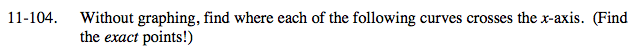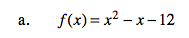Factor.
f(x) = (x + 3)(x − 4)

(−3, 0) and (4, 0)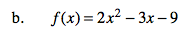(−1.5, 0) and (3, 0)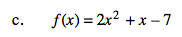See part (b).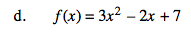See part (b).

It does not intersect the x-axis.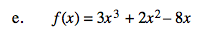x is a common factor.

After you factor out x, factor or use the quadratic formula to find the roots of the rest.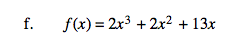See part (e).